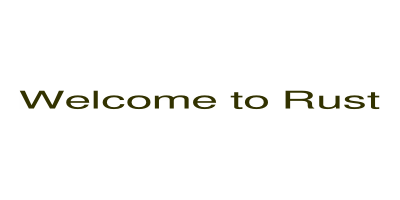# Create image with text written on it using Rust

In this example we create a new image with white background and the we write some text on it centered both horizontally and vertically.

This is the result:## Find the fonts on your computer

First we need to find and select a font that we have on our system:

For example on Linux or macOS you can use either the `locate` command:

``````locate "*.ttf"
``````

or the `find` command

``````find / -name "*.ttf" 2> /dev/null
``````

to find True Type fonts.

On Windows I guess you'd use the File-explorer to find font files.

We could include the font where it is on our system, but it is probably a good idea to copy the file containing the selected font to the folder of the crate so they will be available on any system.

## Crates

We are using the following crates:

examples/create-image-with-text/Cargo.toml

``````[package]
name = "create-image-with-text"
version = "0.1.0"
edition = "2021"

# See more keys and their definitions at https://doc.rust-lang.org/cargo/reference/manifest.html

[dependencies]
image = "0.24"
imageproc = "0.23"
rusttype = "0.9"

``````

## Source code

examples/create-image-with-text/src/main.rs

``````use image::{Rgb, RgbImage};
use imageproc::drawing::{draw_text_mut, text_size};
use rusttype::{Font, Scale};
use std::env;
use std::path::Path;

fn main() {
let argv = env::args().collect::<Vec<String>>();
if argv.len() != 3 {
eprintln!("Usage: {} FILENAME TEXT", &argv);
std::process::exit(1);
}
let path = Path::new(&argv);
let text = &argv;

let width = 400;
let height = 200;

// create image
let mut image = RgbImage::new(width, height);
// set white background
for x in 0..width {
for y in 0..height {
*image.get_pixel_mut(x, y) = image::Rgb([255, 255, 255]);
}
}

//let font = Vec::from(include_bytes!("/usr/share/fonts/truetype/dejavu/DejaVuSerif.ttf") as &[u8]);
//let font = Vec::from(include_bytes!("/snap/cups/980/usr/share/fonts/truetype/freefont/FreeSans.ttf") as &[u8]);
let font = Vec::from(include_bytes!("../FreeSans.ttf") as &[u8]);
let font = Font::try_from_vec(font).unwrap();

let inteded_text_height = 24.4;
let scale = Scale {
x: inteded_text_height * 2.0,
y: inteded_text_height,
};

// color of the text
let red = 50 as u8;
let green = 50;
let blue = 0;

// get the size of the text and calculate the x, y coordinate where to start to be center aligned
// both horizontally and vertically
let (text_width, text_height) = text_size(scale, &font, text);
println!("Text size: {}x{}", text_width, text_height);
let text_start_x = ((width-text_width as u32) / 2 ) as i32;
let text_start_y = ((height-text_height as u32) / 2 ) as i32;

draw_text_mut(&mut image, Rgb([red, green, blue]), text_start_x, text_start_y, scale, &font, text);

image.save(path).unwrap();
}
``````
• First we expect and accept two parameters on the command line. The name of the image file and the text we would like to write on the image.

• Then we create the image and set all the pixels to be white.

• Then we create the font using the include_bytes! macro that embeds the content of the font file in the binary `rustc` compiles.

• Then we calculate the scale of the text and using the `text_size` function we compute the real size of the text taking into account the font that we'll use.

• Based on these and the dimension of the image we calculate the x,y point where the text should start in order to be in the center of the image.

• We then draw the text using the `draw_text_mut` function.

• Finally we save the image to the file.

## Running on the command line during development

We provide the name of the file we would like to create and the text we would like to write on the image.

``````cargo run -- image.png "Welcome to Rust"
``````

## Running on the command line

We can now create a stand-alone binary from our code:

``````cargo build --relase
``````

Then we can distribute the file from `target/release/create-image-with-text`.

The users will be able to run it as

``````create-image-with-text image.png "Hello world!"
``````

The font is already embedded in the binary so we don't need to worry about that.